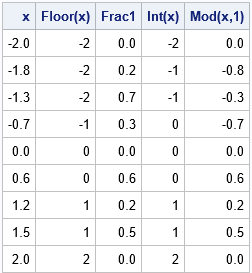You can represent every number as a nearby integer plus a decimal. For example, 1.3 = 1 + 0.3. The integer is called the integer part of x, whereas the decimal is called the fractional part of x (or sometimes the decimal part of x). This representation is not unique. For example, you can also write 1.3 = 2 + (-0.7). There are several ways to produce the integer part of a number, depending on whether you want to round up, round down, round towards zero, or use some alternative rounding method.

Just as each rounding method defines the integer part of a number, so, too, does it define the fractional part. If [x]denotes the integer part of x (by whatever rounding method you choose), then the fractional part is defined by frac(x) = x - [x]. For some choices of a rounding function (for example, FLOOR), the fractional part is positive for all x. For other choices, the fractional part might vary according to the value of x.

In applications, two common representations are as follows:

• Round x towards negative infinity. The fractional part of x is always positive. You can round towards negative infinity by using the FLOOR function in a computer language. For example, if x = -1.3, then FLOOR(x) is -2 and the fractional part is 0.7.
• Round x towards zero. The fractional part of x always has the same sign as x. You can round towards zero by using the INT function. For example, if x = -1.3, then INT(x) is -1 and the fractional part is -0.3.

Here is an interesting fact: for the second method (INT), you can compute the fractional part directly by using the MOD function in SAS. In SAS, the expression MOD(x,1) returns the signed fractional part of a number because the MOD function in SAS returns a result that has the same sign as x. This can be useful when you are interested only in the fraction portion of a number.

The following DATA step implements both common methods for representing a number as an integer and a fractional part. Notice the use of the MOD function for the second method:

```data Fractional; input x @@; /* Case 1: x = floor(x) + frac1(x) where frac1(x) >= 0 */ Floor = floor(x); Frac1 = x - Floor; /* always positive */   /* Case 2: x = int(x) + frac2(x) where frac1(x) has the same sign as x */ Int = int(x); Frac2 = mod(x,1); /* always same sign as x */ label Floor = 'Floor(x)' Int='Int(x)' Frac2='Mod(x,1)'; datalines; -2 -1.8 -1.3 -0.7 0 0.6 1.2 1.5 2 ;   proc print data=Fractional noobs label; run;```The table shows values for a few positive and negative values of x. The second and third columns represent the number as x = Floor(x) + Frac1. The fourth and fifth columns represent the number as x = Int(x) + Mod(x,1). For non-negative values of x, the two methods are equivalent. When x can be either positive or negative, I often find that the second representation is easier to work with.

In statistics, the fractional part of a number is used in the definition of sample estimates for percentiles and quantiles. SAS supports five different definitions of quantiles, some of which look at the fractional part of a number to decide how to estimate a percentile.

Share1.